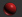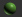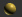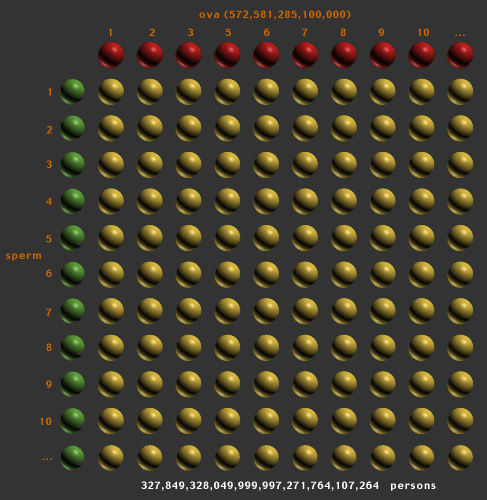```e v e r y  -  p e r s o n
```

The number of possible persons is determined by combining the current world supply of ova with the world supply of sperm.

 person = combination of specific ovum and sperm current population = 6240272424 personsaverage age of puberty (menarche) in female = 12.8 years average age of menopause in female = 50.5 years average number of years female releases ova = 37.7 years ova released by female per year = 13.04 ova per year average number of ova released over lifetime of female = 491.77 ova average number of ova produced over lifetime of female (a) = 400000 ova current population of females 15 to 65 years old = 1898479062 current population of females 12.8 to 50.5 years old capable of reproduction (f) = 1431453213 number of ova available from current population (a x f) = 572581285100000 ovaaverage age of puberty in male = 12.5 years average age of male menopause = 55 years average number of years male produces sperm = 42.5 years average number of sperm cells per ejaculate = 400000000 sperm average number of ejaculates per year from 15 year old male = 152.08 average number of ejaculates per year from 55 year old male = 43.5 average number of ejaculates per year during fertile life of male = 97.79 average number of sperm ejaculated during fertile life of male = 39116666667 sperm per year average number of sperm ejaculated over lifetime of male (b) = 1662458333300 sperm current number of males 15-65 years old = 1942402264 current number of males 12.5 to 55 years old capable of reproduction (m) = 1651041924 number of sperm available from current population (b x m) = 2744788405899999838208number of ova needed to create person = 1 ovum number of sperm required to create person = 1 sperm 1 ovum + 1 sperm = c = 2 available supply of ova = v = 572581285100000 ova available supply of sperm > available supply of ova

 e = v^cevery-person = e = 572581285100000^2 possible personsworld population relative to number of possible persons = (100 x 6240272424 / 327849328049999997271764107264) %